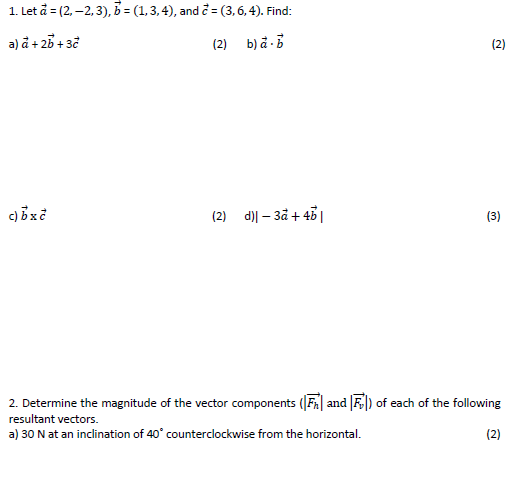# Question Solved1 Answer 1. Let à = (2,-2,3), b = (1,3,4), and = (3,6,4). Find: a) a + 2b +3c (2) b) a-b c) bxc (2) d)| -3a + 4b | (3) 2. Determine the magnitude of the vector components (F and F) of each of the following resultant vectors. a) 30 N at an inclination of 40° counterclockwise from the horizontal. (2) (2) 1. Let à = (2,-2,3), b = (1,3,4), and = (3,6,4). Find: a) a + 2b +3c (2) b) a-b c) bxc (2) d)| -3a + 4b | (3) 2. Determine the magnitude of the vector components (F and F) of each of the following resultant vectors. a) 30 N at an inclination of 40° counterclockwise from the horizontal. (2) (2)Transcribed Image Text: 1. Let à = (2,-2,3), b = (1,3,4), and = (3,6,4). Find: a) a + 2b +3c (2) b) a-b c) bxc (2) d)| -3a + 4b | (3) 2. Determine the magnitude of the vector components (F and F) of each of the following resultant vectors. a) 30 N at an inclination of 40° counterclockwise from the horizontal. (2) (2)
More
Transcribed Image Text: 1. Let à = (2,-2,3), b = (1,3,4), and = (3,6,4). Find: a) a + 2b +3c (2) b) a-b c) bxc (2) d)| -3a + 4b | (3) 2. Determine the magnitude of the vector components (F and F) of each of the following resultant vectors. a) 30 N at an inclination of 40° counterclockwise from the horizontal. (2) (2)